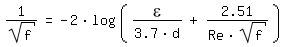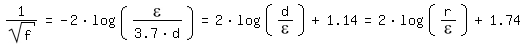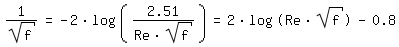﻿﻿﻿ Pressure loss Straight Piping ﻿ ﻿ ﻿ ﻿

# Pressure loss of the viscous flow through straight pipes

 Δ p = f · l/d · ρ/2 · v2       (Darcy-Weisbach formula)
 dimensioned variables (metric as example) Δ p [kg/(m s²) = 10-5 bar = 10-2mbar] : Pressure loss of the straight pipe l [m] : Length d [m] : Internal Diameter ρ [kg/m³] : Density v [m/s] : Average speed in the pipe η [kg/(m s) = Pas] : Dyn. Viscosity ε [m] : Roughness dimensionless values Re [-] : Reynolds number Re = ρ v d / η f [-] : Darcy Friction Factor

For Re < 2320 (laminar flow) the Darcy Friction Factor is calculated according to Hagen-Poiseuille:

f = 64 / ReHagen Poiseuille and Couette Flow

For Re > 2320 (turbulent flow) the Darcy Friction Factor is calculated according to [Colebrook 1939, page 137]:For Re => ∞ (completely turbulent flow) the equation of Colebrook converges to the equation of [Prandtl 1933, page 110] according to Kármán:and for ε/d => 0 (smooth pipe) to the equation of [Prandtl 1933, page 111]:Laminar flow is possible for Re < 8000 [VDI 1984, page Lb1]. In the critical transition zone (2320 <= Re < 8000) the value of the Darcy Friction Factor might be overestimated by the means of calculation presented above.

The Moody-diagram [Moody 1944, page 672] is a successful illustration of the equations presented above.MoodyRe: 10-106 MoodyRe: 100-108

With 1/f^0,5 = Re/200 · ε /d   and    1/f^0,5 = 2log(r/ε) + 1,74   [Moody 1944, page 676] draws a line between the transition zone and the completely turbulent pipe flow.

 Literature [Colebrook 1939] C.F. Colebrook, Turbulent Flow in Pipes, with particular reference to the Transition Region between the Smooth and Rough Pipe Laws, Journal of The Institution of Civil Engineers, Volume 11, 1939 [Moody 1944] L.F.Moody, Friction Factors of Pipe Flow, Transactions of the ASME, Volume 66, 1944 [Prandtl 1933] L. Prandtl, Neuere Ergebnisse der Turbulenzforschung, Zeitschrift des Vereins Deutscher Ingenieure, Band 77, 1933 [VDI 1984] VDI-Wärmeatlas 4. Auflage 1984

﻿